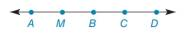Chapter 1.3, Problem 15E### Elementary Geometry for College St...

6th Edition
Daniel C. Alexander + 1 other
ISBN: 9781285195698

#### Solutions

Chapter
Section### Elementary Geometry for College St...

6th Edition
Daniel C. Alexander + 1 other
ISBN: 9781285195698
Textbook Problem
1 views

# In Exercises 9 to 28, use the drawings as needed to answer the following questions.Exercises 14 − 17 Given: M is the midpoint of A B ¯ A M = 2 x + 1 and M B = 3 x − 2 Find: x and A M

To determine

To find:

The values of x and AM.

Explanation

Given:

The given figure is,

M is the midpoint of AB¯.

AM=2x+1 and MB=3x2.

Calculation:

Consider the given figure,

Figure (1)

M is the midpoint of AB¯.

AM¯=MB¯

Also, AM=2x+1 and MB=3x2.

### Still sussing out bartleby?

Check out a sample textbook solution.

See a sample solution

#### The Solution to Your Study Problems

Bartleby provides explanations to thousands of textbook problems written by our experts, many with advanced degrees!

Get Started

#### Find more solutions based on key concepts